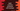# Swift 4 tutorial : continue statementSuppose your program has a loop ( for or while ) and you want to skip some specific conditions, i.e. you don’t want to run the code inside the loop for these conditions. ‘continue’ statement is used for that. It informs the loop to stop for that iteration and continue from the next iteration. In this tutorial , we will check different use cases of ‘continue’. ’ The syntax used for ‘continue’ is :

``````continue
``````

### Example of continue statement with a for loop in swift :

Check the below example program :

``````for i in 0...15{
if(i < 10){
continue
}
print ("i = \(i)")
}
``````

Output :

``````i = 10
i = 11
i = 12
i = 13
i = 14
i = 15
``````

The above ‘for’ loop will run from i=0 to i=15. On each iteration, it will print the value of ‘i’ . But if i is less than 10, we have one ‘continue’ statement i.e. the loop will return from this line and continue the next iteration. That means for i = 1,2,3,4,5,6,7,8 and 9 , it will not print anything. Only from i = 10 , the loop will start printing.

### Example of swift continue statement with a while loop :

Example Program :

``````var value = 15

while (value > 1){
if(value % 2 == 0){
value = value - 1
continue
}
print ("Current value is = \(value)")
value = value - 1
}
``````

Output :

``````Current value is = 15
Current value is = 13
Current value is = 11
Current value is = 9
Current value is = 7
Current value is = 5
Current value is = 3
``````

It worked same as ‘for’ loop. The ‘while’ loop starts from ‘value = 15’ and it will continue till ‘value > 1’ i.e. it will run from ‘value = 15’ to ‘value = 2’ : 14 times. Inside the loop, we are printing out the current value only if it is not divisible by 2. If ‘value’ is divisible by 2, decrease the value by 1 and ‘continue’ the loop .

### Using ‘continue’ in swift nested for loop:

We can also use ‘continue’ statement with nested for loop in swift. The ‘continue’ will tell the same ‘for loop’ to stop execution where you will use it. For example :

``````for i in 1...5 {
if( i%2 != 0){
continue
}
for j in 1...5{
if( j%2 != 0){
continue
}
print ("i | j = \(i) | \(j)")
}
}
``````

It will give the following output :

``````i | j = 2 | 2
i | j = 2 | 4
i | j = 4 | 2
i | j = 4 | 4
``````

In this example, the outer for loop runs only for even numbers i.e. only if ‘i’ is even. Similarly, the inner loop also runs only if ‘j’ is even. That means the ‘print’ statement runs only if ‘i’ and ‘j’ both are even.

### Using labeled for loop with continue :

We can add one label for a for loop. You can think it as name for that loop. Now if we use ‘continue label-name’ , where ‘label-name’ is the name for a for loop label, the control will move directly to the for-loop with that label. Let me explain to you with an example :

``````outerloop: for i in 1...5 {
if( i%2 != 0){
continue
}
innerloop: for j in 1...5{
if( j == 3){
continue outerloop
}
print ("i | j = \(i) | \(j)")
}
}
``````

In this example, ‘outerloop’ is the label for outer for-loop and ‘innerloop’ is the label for inner for-loop. ‘continue outerloop’ statement moves the control to the beginning of ‘outerloop’ i.e. the inner for-loop will exit . It produces the following output :

``````i | j = 2 | 1
i | j = 2 | 2
i | j = 4 | 1
i | j = 4 | 2
``````

Each time ‘j’ in the inner loop is equal to 3, it will exit to the outerloop. Also the outerloop runs only if ‘i’ is divisible by 2, otherwise it will ‘continue’.